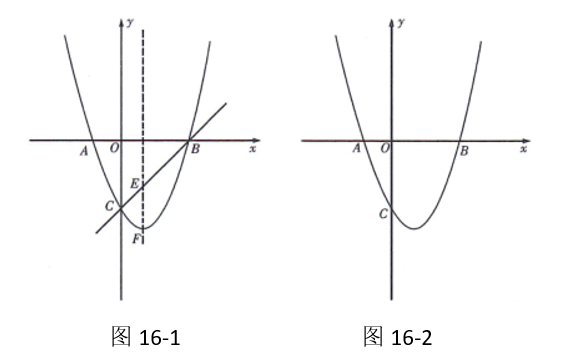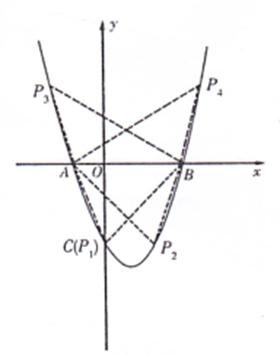(1)求该抛物线的解析式;
(2)若点 $E$ 是抛物线的对称轴与直线 $B C$ 的交点, 点 $F$ 是抛物线的顶点, 求 $E F$ 的长;
(3)设点 $P$ 是(1)中抛物线上的一个动点, 是否存在满足 $\mathrm{S}_{\triangle \mathrm{PAB}}=6$ 的点 $P$ ?如果存 在, 请求出点 P的坐标; 若不存在, 请说明理由.(请在图 16-2 中探讨)【答案】 解: (1) $\because$ 抛物线 $\mathrm{y}=\mathrm{x}^2+\mathrm{bx}+\mathrm{c}$ 与 $\mathrm{x}$ 轴的两个交点分别为 $\mathrm{A}(-1,0), \mathrm{B}(3,0)$,
$\therefore$ 所求抛物线的解析式为 $\mathrm{y}=\mathrm{x}^2-2 \mathrm{x}-3 .$
(2) 由 (1) 知, 抛物线的解析式为 $y=x^2-2 x-3$,

$0=3 \mathrm{k}-3$,

$\therefore E F=|-4|-|-2|=2$, 即 $E F=2$.

(3) 设点 $\mathrm{P}(\mathrm{x}, \mathrm{y})$, 由题意, 得 $S_{\triangle \mathrm{PAB}}=12 \times 4|\mathrm{y}|=6$,
$\therefore|\mathrm{y}|=3, \quad \therefore \mathrm{y}=\pm 3$

$\therefore x_1=0, x_2=2$,

$\therefore \mathrm{x}_3=1-\sqrt{7} \quad \mathrm{x}_4=1+\sqrt{7}$,
$\therefore$ 当点 $P$ 的坐标分别为
$P_1(0,-3), P_2(2,-3), P_3(1-7,3), P_4(1+7,3)$ 时,
$S_{\triangle \mathrm{PAB}}=6$.